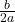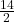## Find the coordinates of the turning point for the equation of the graph below y=x^2+14x+45

Question

Find the coordinates of the turning point for the equation of the graph below y=x^2+14x+45

in progress 0
2 weeks 2021-09-04T21:14:58+00:00 1 Answers 0 views 0

(- 7, – 4 )

Step-by-step explanation:

Given a quadratic in standard form

y = ax² + bx + c ( a ≠ 0 )

Then the x- coordinate of the turning point is

x = –y = x² + 14x + 45 ← is in standard form

with a = 1, b = 14 , then

x = –= – 7

Substitute x = – 7 into the equation and evaluate for y

y = (- 7)² + 14(- 7) + 45 = 49 – 98 + 45 = – 4

coordinates of turning point = (- 7, – 4 )